# Resources tagged with: Triangles

Filter by: Content type:
Age range:
Challenge level:

### There are 57 results

Broad Topics > Angles, Polygons, and Geometrical Proof > Triangles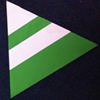### Regular Rings 1

##### Age 7 to 11Challenge Level

Can you work out what shape is made by folding in this way? Why not create some patterns using this shape but in different sizes?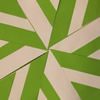### Folding Flowers 2

##### Age 7 to 11Challenge Level

Make a flower design using the same shape made out of different sizes of paper.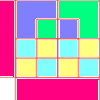### Diagrams

##### Age 7 to 11Challenge Level

A group activity using visualisation of squares and triangles.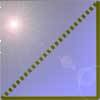### Cutting Corners

##### Age 7 to 11Challenge Level

Can you make the most extraordinary, the most amazing, the most unusual patterns/designs from these triangles which are made in a special way?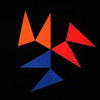### Notes on a Triangle

##### Age 11 to 14Challenge Level

Can you describe what happens in this film?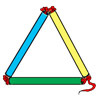### Making Maths: Test the Strength of a Triangle

##### Age 7 to 11Challenge Level

Have you noticed that triangles are used in manmade structures? Perhaps there is a good reason for this? 'Test a Triangle' and see how rigid triangles are.### Cut and Make

##### Age 7 to 11Challenge Level

Cut a square of paper into three pieces as shown. Now,can you use the 3 pieces to make a large triangle, a parallelogram and the square again?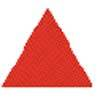### Tessellating Triangles

##### Age 7 to 11Challenge Level

Can you make these equilateral triangles fit together to cover the paper without any gaps between them? Can you tessellate isosceles triangles?### Rectangle Tangle

##### Age 7 to 11Challenge Level

The large rectangle is divided into a series of smaller quadrilaterals and triangles. Can you untangle what fractional part is represented by each of the shapes?### Cutting it Out

##### Age 5 to 11Challenge Level

I cut this square into two different shapes. What can you say about the relationship between them?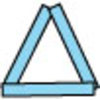### Sticks and Triangles

##### Age 7 to 11Challenge Level

Using different numbers of sticks, how many different triangles are you able to make? Can you make any rules about the numbers of sticks that make the most triangles?### Putting Two and Two Together

##### Age 7 to 11Challenge Level

In how many ways can you fit two of these yellow triangles together? Can you predict the number of ways two blue triangles can be fitted together?### Constructing Triangles

##### Age 11 to 14Challenge Level

Generate three random numbers to determine the side lengths of a triangle. What triangles can you draw?### Inside Seven Squares

##### Age 7 to 11Challenge Level

What is the total area of the four outside triangles which are outlined in red in this arrangement of squares inside each other?### Tri.'s

##### Age 7 to 11Challenge Level

How many triangles can you make on the 3 by 3 pegboard?### More Transformations on a Pegboard

##### Age 7 to 11Challenge Level

Use the interactivity to find all the different right-angled triangles you can make by just moving one corner of the starting triangle.### Uncanny Triangles

##### Age 7 to 11Challenge Level

Can you help the children find the two triangles which have the lengths of two sides numerically equal to their areas?### Name That Triangle!

##### Age 7 to 11Challenge Level

Can you sketch triangles that fit in the cells in this grid? Which ones are impossible? How do you know?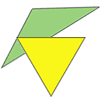### Triangle Relations

##### Age 7 to 11Challenge Level

What do these two triangles have in common? How are they related?### Bracelets

##### Age 7 to 11Challenge Level

Investigate the different shaped bracelets you could make from 18 different spherical beads. How do they compare if you use 24 beads?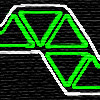### Tri-five

##### Age 7 to 11Challenge Level

Find all the different shapes that can be made by joining five equilateral triangles edge to edge.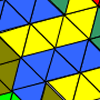### Tessellating Transformations

##### Age 7 to 11Challenge Level

Can you find out how the 6-triangle shape is transformed in these tessellations? Will the tessellations go on for ever? Why or why not?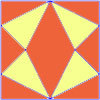### Fraction Fascination

##### Age 7 to 11Challenge Level

This problem challenges you to work out what fraction of the whole area of these pictures is taken up by various shapes.### Nine-pin Triangles

##### Age 7 to 11Challenge Level

How many different triangles can you make on a circular pegboard that has nine pegs?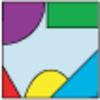### Jig Shapes

##### Age 5 to 11Challenge Level

Can you each work out what shape you have part of on your card? What will the rest of it look like?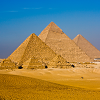### Egyptian Rope

##### Age 7 to 11Challenge Level

The ancient Egyptians were said to make right-angled triangles using a rope with twelve equal sections divided by knots. What other triangles could you make if you had a rope like this?### Folding

##### Age 7 to 11Challenge Level

What shapes can you make by folding an A4 piece of paper?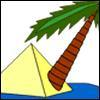### Triangle Island

##### Age 7 to 11Challenge Level

You have pitched your tent (the red triangle) on an island. Can you move it to the position shown by the purple triangle making sure you obey the rules?### Counting Triangles

##### Age 11 to 14Challenge Level

Triangles are formed by joining the vertices of a skeletal cube. How many different types of triangle are there? How many triangles altogether?### Cut it Out

##### Age 7 to 11Challenge Level

Can you dissect an equilateral triangle into 6 smaller ones? What number of smaller equilateral triangles is it NOT possible to dissect a larger equilateral triangle into?### Possible Pairs

##### Age 7 to 11Challenge Level

In this game, you turn over two cards and try to draw a triangle which has both properties.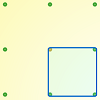### Peg and Pin Boards

##### Age 5 to 11

This article for teachers suggests activities based on pegboards, from pattern generation to finding all possible triangles, for example.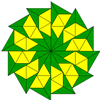### Triangle Shapes

##### Age 5 to 11Challenge Level

This practical problem challenges you to create shapes and patterns with two different types of triangle. You could even try overlapping them.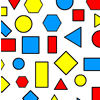### Sorting Logic Blocks

##### Age 5 to 11Challenge Level

This activity focuses on similarities and differences between shapes.### Making Maths: Equilateral Triangle Folding

##### Age 7 to 14Challenge Level

Make an equilateral triangle by folding paper and use it to make patterns of your own.### Triangle Pin-down

##### Age 7 to 11Challenge Level

Use the interactivity to investigate what kinds of triangles can be drawn on peg boards with different numbers of pegs.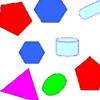### Part the Polygons

##### Age 7 to 11 ShortChallenge Level

Draw three straight lines to separate these shapes into four groups - each group must contain one of each shape.### Triangles All Around

##### Age 7 to 11Challenge Level

Can you find all the different triangles on these peg boards, and find their angles?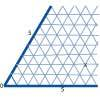### Cartesian Isometric

##### Age 7 to 11Challenge Level

The graph below is an oblique coordinate system based on 60 degree angles. It was drawn on isometric paper. What kinds of triangles do these points form?### Tetrahedra Tester

##### Age 11 to 14Challenge Level

An irregular tetrahedron is composed of four different triangles. Can such a tetrahedron be constructed where the side lengths are 4, 5, 6, 7, 8 and 9 units of length?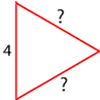### Number the Sides

##### Age 7 to 11Challenge Level

The triangles in these sets are similar - can you work out the lengths of the sides which have question marks?### Triangular Tantaliser

##### Age 11 to 14Challenge Level

Draw all the possible distinct triangles on a 4 x 4 dotty grid. Convince me that you have all possible triangles.### Isosceles Triangles

##### Age 11 to 14Challenge Level

Draw some isosceles triangles with an area of $9$cm$^2$ and a vertex at (20,20). If all the vertices must have whole number coordinates, how many is it possible to draw?### Squareo'scope Determines the Kind of Triangle

##### Age 11 to 14

A description of some experiments in which you can make discoveries about triangles.### Property Chart

##### Age 11 to 14Challenge Level

A game in which players take it in turns to try to draw quadrilaterals (or triangles) with particular properties. Is it possible to fill the game grid?### Shapely Pairs

##### Age 11 to 14Challenge Level

A game in which players take it in turns to turn up two cards. If they can draw a triangle which satisfies both properties they win the pair of cards. And a few challenging questions to follow...### Transformations on a Pegboard

##### Age 7 to 11Challenge Level

How would you move the bands on the pegboard to alter these shapes?### Trice

##### Age 11 to 14Challenge Level

ABCDEFGH is a 3 by 3 by 3 cube. Point P is 1/3 along AB (that is AP : PB = 1 : 2), point Q is 1/3 along GH and point R is 1/3 along ED. What is the area of the triangle PQR?### LOGO Challenge 5 - Patch

##### Age 11 to 16Challenge Level

Using LOGO, can you construct elegant procedures that will draw this family of 'floor coverings'?### Lighting up Time

##### Age 7 to 14Challenge Level

A very mathematical light - what can you see?